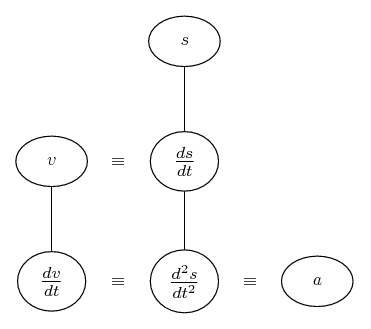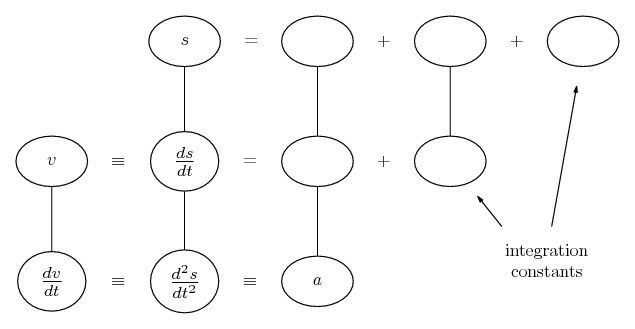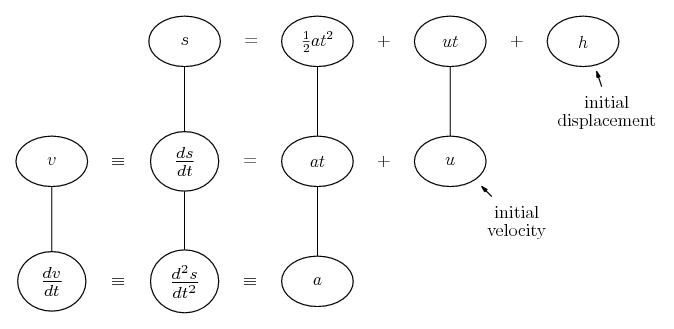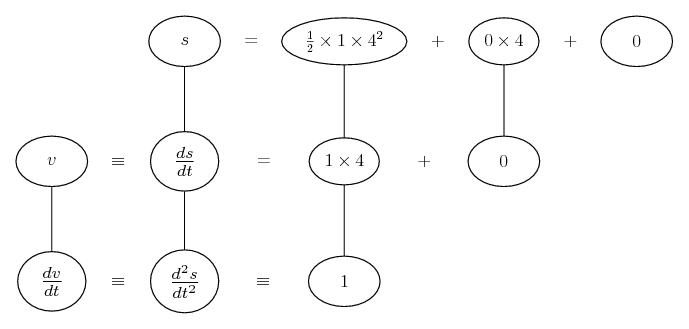# Thread: help needed changing words to formula for integration

1. ##help needed changing words to formula for integration

Hey guys,

I know how to get the answer but I don't know what the formulas areThe questions goes..

You start at rest on a bike. You accelerate at 1 m/s^2 for 4 seconds and then travel at a constant velocity until you arrive at your destination which is 80 metres from the starting position. Calculate travelling time.

I know I have to integrate twice to find the distance traveled during the acceleration period but I don't know what the formula for this question will be. I have a(t) = t ? But I don't get the correct answer when I integrate..

I can do the question in my head easy but we have to use integration.

Thanks

2. Why can't you use the kinematic equations to solve this problem? Is there any instruction to solve this problem by integration?

3. Just in case a picture helps, and if you do want or need to derive the formulae by integration from the definitions of velocity v and acceleration a in terms of displacement, s ...... where straight lines are differentiating downwards with respect to t. Well, integrating a twice with respect to t ...Spoiler:Spoiler:So after 4 seconds the displacement is 8, and you've 72 metres to go, at 4 m/s.

_________________________________________

Don't integrate - balloontegrate!

Balloon Calculus; standard integrals, derivatives and methods

Balloon Calculus Drawing with LaTeX and Asymptote!

4. Its for a calculus course I am doing so I just assumed they would want me to use calculus to find the answer.

5.Originally Posted by smpleaseHey guys,

I know how to get the answer but I don't know what the formulas areThe questions goes..

You start at rest on a bike. You accelerate at 1 m/s^2 for 4 seconds and then travel at a constant velocity until you arrive at your destination which is 80 metres from the starting position. Calculate travelling time.

I know I have to integrate twice to find the distance traveled during the acceleration period but I don't know what the formula for this question will be. I have a(t) = t ? But I don't get the correct answer when I integrate..

I can do the question in my head easy but we have to use integration.

Thanks
I suggest that you start by drawing a velocity-time graph.

6. No, a(t) is not t. You are told that the acceleration is 1 m/s^2 for 4 seconds- a(t)= 1. What is the velocity function at each time t for those 4 seconds? What will your velocity be at the end of the 4 seconds? What will your position be at the end of the 4 seconds? What distance do you still have to go to get to a total of 80 m? How long will that take at your new velocity?

7. So during the period of acceleration.
v = t and will be 4 m/s at the end of the period of acceleration.
Displacement will be s = t^2/2. So displacement after 4 seconds will be 8 metres?
Leaving 72 metres left to travel at constant velocity of 4 m/s, which would take 18 seconds and then add the time taken in the acceleration period of 4 seconds to get 22 seconds all up?

8. Correct.Were you aware of the function of the 'spoiler' button, by the way?

#### Search Tags

changing, formula, integration, needed, words